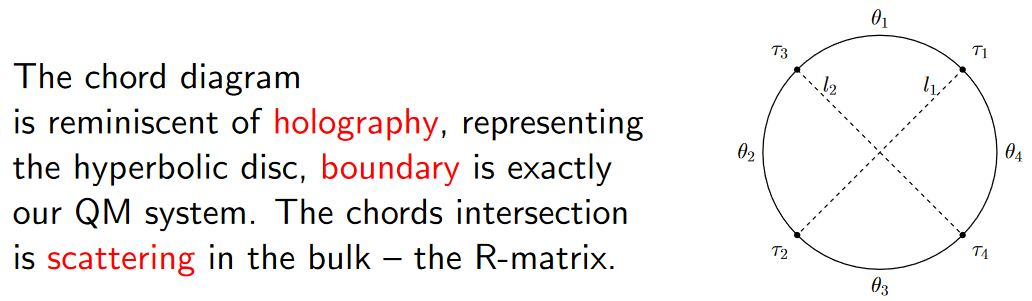Contents

# Contents

## Idea

Studies of holography have been hampered by the lack of a simple solvable model that can capture features of Einstein gravity. The simplest model, which is a single matrix quantum mechanics, does not appear to lead to black holes. $\mathcal{N} = 4$ super Yang Mills at strong ’t Hooft coupling certainly leads to black holes, and exact results are known at large N for many anomalous dimensions and some vacuum correlation functions, but at finite temperature the theory is difficult to study.

A system that reproduces some of the dynamics of black holes should be interacting, but we might hope for a model with interactions that are simple enough that it is still reasonable solvable.

Kitaev has proposed to study a quantum mechanical model of $N$ Majorana fermions interacting with random interactions (Kitaev 15). It is a simple variant of a model introduced by Sachdev and Ye (Sachdev-Ye 93), which was first discussed in relation to holography in (Sachdev 10).

From Maldacena 18:

The SYK model gives us a glimpse into the interior of an extremal black hole… That’s the feature of SYK that I find most interesting… It is a feature this model has, that I think no other model has

## Definition

Let $\mathcal{J}_{i j k l}$ be random variables with expectation values $E[\mathcal{J}_{i j k l}]=0$ and $E[\mathcal{J}_{i j k l}^2]=\frac{6J^2}{N^3}$.

The Lagrangian density defining the SYK model is this:

$L = \frac{1}{2} \sum_{i=1}^N \chi_i \partial_t \chi_i - \frac{1}{4!} \sum_{i,j,k,l=1}^N \mathcal{J}_{i j k l} \chi_i \chi_j \chi_k \chi_l$

### General

The model is due to

Textbook accounts:

Further review:

Further developments:

Relation to random matrix theory:

• Yiyang Jia, Jacobus J. M. Verbaarschot, Spectral Fluctuations in the Sachdev-Ye-Kitaev Model (arXiv:1912.11923)

Discussion of possible realization of the SYK-model in condensed matter physics:

• D. I. Pikulin, M. Franz, Black hole on a chip: proposal for a physical realization of the SYK model in a solid-state system, Phys. Rev. X 7, 031006 (2017) (arXiv:1702.04426)

Relation of the SYK-model to the strange metals via AdS/CMT:

### SYK-model in $AdS_2/CFT_1$

Discussion of the SYK-model as the AdS/CFT dual of JT-gravity in nearly AdS2/CFT1 and AdS-CFT in condensed matter physics:

Original articles:

Review:

Relation to black holes in terms of Majorana dimer states:

• Ioanna Kourkoulou, Juan Maldacena, Pure states in the SYK model and nearly-$AdS_2$ gravity (arXiv:1707.02325)

Relation to black holes in string theory and random matrix theory:

• Yuri D. Lensky, Xiao-Liang Qi, Pengfei Zhang, Size of bulk fermions in the SYK model (arXiv:2002.01961)

• Xiao-Liang Qi, Pengfei Zhang, The Coupled SYK model at Finite Temperature (arXiv:2003.03916)

• Akash Goel, Herman Verlinde, Towards a String Dual of SYK (arXiv:2103.03187)

• Tarek Anous, Felix M. Haehl, The quantum $p$-spin glass model: A user manual for holographers (arXiv:2106.03838)

### In terms of chord diagrams

Discussion of (Lie algebra-)weight systems on chord diagrams encoding SYK model single trace observables:

(for more see at weight systems on chord diagrams in physics)

• Antonio M. García-García, Yiyang Jia, Jacobus J. M. Verbaarschot, Exact moments of the Sachdev-Ye-Kitaev model up to order $1/N^2$, JHEP 04 (2018) 146 (arXiv:1801.02696)

• Yiyang Jia, Jacobus J. M. Verbaarschot, Section 4 of: Large $N$ expansion of the moments and free energy of Sachdev-Ye-Kitaev model, and the enumeration of intersection graphs, JHEP 11 (2018) 031 (arXiv:1806.03271)

• Micha Berkooz, Prithvi Narayan, Joan Simón, Chord diagrams, exact correlators in spin glasses and black hole bulk reconstruction, JHEP 08 (2018) 192 (arxiv:1806.04380)

following:

• László Erdős, Dominik Schröder, Phase Transition in the Density of States of Quantum Spin Glasses, D. Math Phys Anal Geom (2014) 17: 9164 (arXiv:1407.1552)

which in turn follows

• Philippe Flajolet, Marc Noy, Analytic Combinatorics of Chord Diagrams, pages 191–201 in Daniel Krob, Alexander A. Mikhalev,and Alexander V. Mikhalev, (eds.), Formal Power Series and Algebraic Combinatorics, Springer 2000 (doi:10.1007/978-3-662-04166-6_17)With emphasis on holographic content:

• Micha Berkooz, Mikhail Isachenkov, Vladimir Narovlansky, Genis Torrents, Section 5 of: Towards a full solution of the large $N$ double-scaled SYK model, JHEP 03 (2019) 079 (arxiv:1811.02584)

• Vladimir Narovlansky, Slide 23 (of 28) of: Towards a Solution of Large $N$ Double-Scaled SYK, 2019 (pdf)

and specifically in relation to Jackiw-Teitelboim gravity: